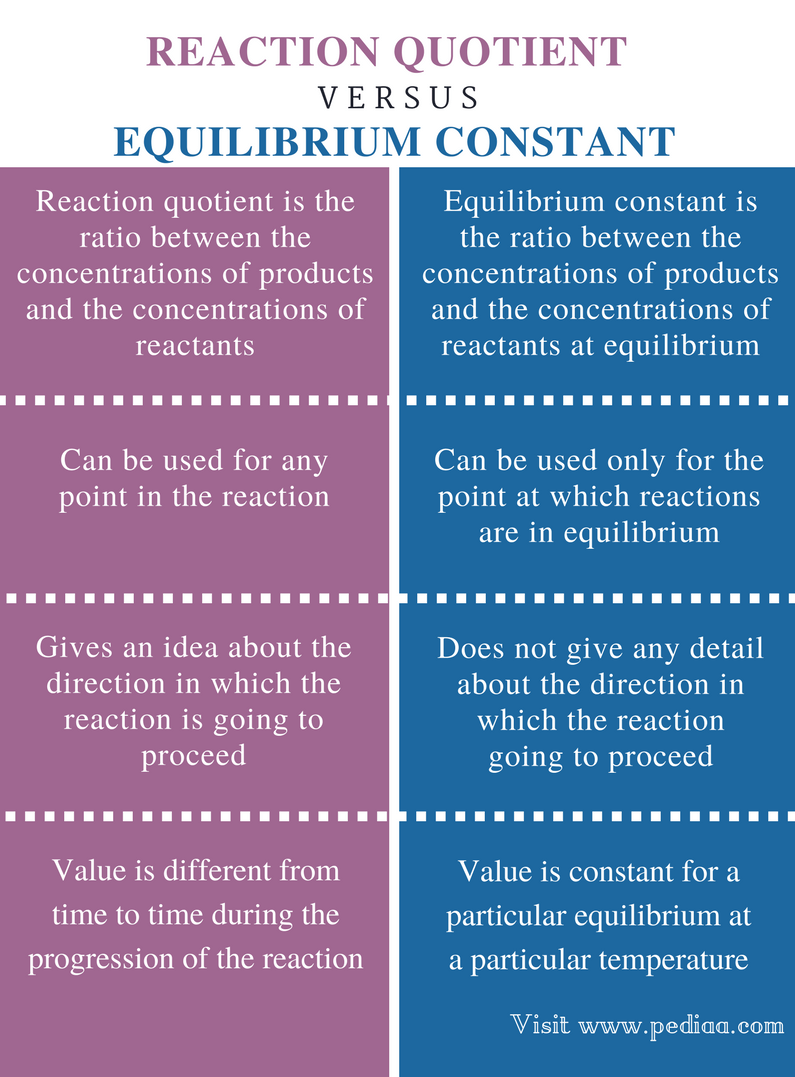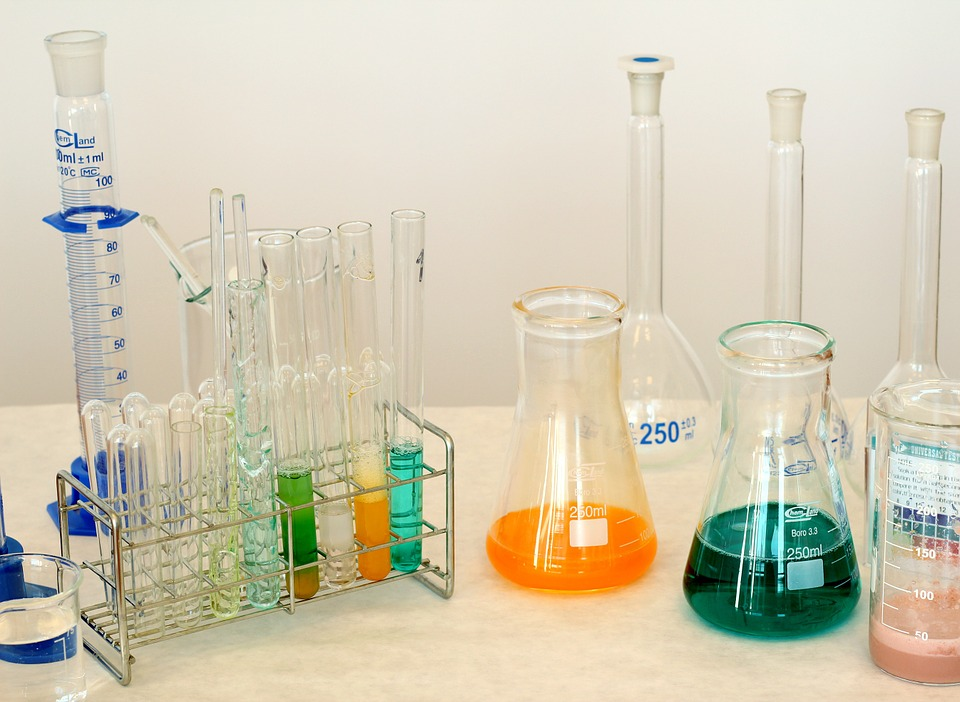# Difference Between Reaction Quotient and Equilibrium Constant

## Main Difference – Reaction Quotient vs Equilibrium Constant

All chemical reactions that happen in a system can be recognized as either equilibrium reactions or non-equilibrium reactions. A reaction becomes an equilibrium reaction when the reactants are not completely dissociated into their ions. Non-equilibrium reactions include the complete ionization of reactants. Reaction quotient and equilibrium constant are two terms used to explain the chemical reactions occur in a system. Reaction quotient gives an idea about the amounts of chemical species present in a reaction mixture. Equilibrium constant is the ratio between the concentrations of products and the concentrations of reactants. The main difference between reaction quotient and equilibrium constant is that reaction quotient can be calculated for a reaction at any time whereas equilibrium constant is calculated at the point of equilibrium.

### Key Areas Covered

1. What is Reaction Quotient

– Definition, Equation for Calculation, Examples
2. What is Equilibrium Constant

– Definition, Application, Examples

3. What is the Relationship Between Reaction Quotient and Equilibrium Constant

– Correlations explained
4.
What is the Difference Between Reaction Quotient and Equilibrium Constant

– Comparison of Key Differences

Key Terms: Equilibrium, Equilibrium Constant, Ionization, Reactants, Reaction Quotient, Stoichiometry## What is Reaction Quotient

Reaction quotient is the ratio between the concentrations of products and the concentrations of reactants. This can be abbreviated mathematically as below. Let us consider the following reaction.

N2 (g)   +   3H2 (g)           ↔          2NH3 (g)

The reaction quotient for this reaction can be given as below. When writing the reaction quotient, one should also consider the stoichiometry of the components. Here, the stoichiometric coefficient that shows the ratio of the components is also considered. The concentration is raised to the power of that coefficient.

The reaction quotient for the above reaction is,

Reaction Quotient (Qc)  =       [NH3 (g)] 2 / [N2 (g)] [H2 (g)] 3

The reaction quotient can be calculated at any time of the reaction. This means, the reaction quotient of a system can be calculated for a reaction before it reaches the equilibrium, when a change in equilibrium has been done or when the reaction is in equilibrium.

Instead of the concentration of the components, the “activity” of each component can also be used to calculate the reaction quotient. The activity of a substance describes the chemical potential of that substance.## What is Equilibrium Constant

Equilibrium constant is the ratio between the concentrations of products and the concentrations of reactants at equilibrium. This term is used only with reactions that are in equilibrium. The reaction quotient and the equilibrium constant are the same for reactions that are in equilibrium.

The equilibrium constant is also given as the concentrations raised to the power of stoichiometric coefficients. The equilibrium constant is dependent on the temperature of the system considered since the temperature affects the solubility of components and the volume expansion. However, the equation for the equilibrium constant does not include any details about solids that are among the reactants or the products.  Only the substances in liquid phase and gaseous phase are considered.

For example, let us consider the equilibrium between carbonic acid and bicarbonate ion.

H2CO3 (aq)        ↔          HCO3(aq)         +        H+ (aq)

The equilibrium constant for above reaction is given as below.

Equilibrium Constant (K) = [HCO3(aq)] [H+ (aq)] / [H2CO3 (aq)]

## Relationship Between Reaction Quotient and Equilibrium Constant

• If the value of reaction quotient (Q) is higher than that of equilibrium constant (K), the reaction favour reactants more since the amount of products in the system is higher than that of reactants. Then the reaction tends to form more reactants in order to keep the equilibrium at constant.
• If Q is lower than K, the system is composed of more reactants than products. Therefore, the reaction tends to form more products in order to keep the equilibrium.
• If Q and K are equal, then the reaction mixture is at an equilibrium.

## Difference Between Reaction Quotient and Equilibrium Constant

### Definition

Reaction Quotient: Reaction quotient is the ratio between the concentrations of products and the concentrations of reactants.

Equilibrium Constant: Equilibrium constant is the ratio between the concentrations of products and the concentrations of reactants at equilibrium.

### Application

Reaction Quotient: Reaction quotient can be used for any point in the reaction (before it reaches the equilibrium or after).

Equilibrium Constant: Equilibrium constant can be used only for the point at which reactions are in equilibrium.

### Details of Direction

Reaction Quotient: The reaction quotient gives an idea about the direction in which the reaction is going to proceed.

Equilibrium Constant: The equilibrium constant does not give any detail about the direction in which the reaction going to proceed.

### Value

Reaction Quotient: The value of reaction quotient is different from time to time during the progression of the reaction.

Equilibrium Constant: The value of the equilibrium constant is constant for a particular equilibrium at a particular temperature.

### Conclusion

There is a distinct difference between reaction quotient and equilibrium constant although both look the same. This is because the reaction quotient includes the concentration of components at any point of the reaction whereas the equilibrium constant includes the concentrations of each component at the equilibrium. Therefore, it is very important to use the correct details for each term of these reactions.

##### References:

1.”The Reaction Quotient.” Chemistry LibreTexts. Libretexts, 09 Apr. 2017. Web. Available here. 13 July 2017.

##### Image Courtesy:

1. “1009178” (Public Domain) via Pixabay